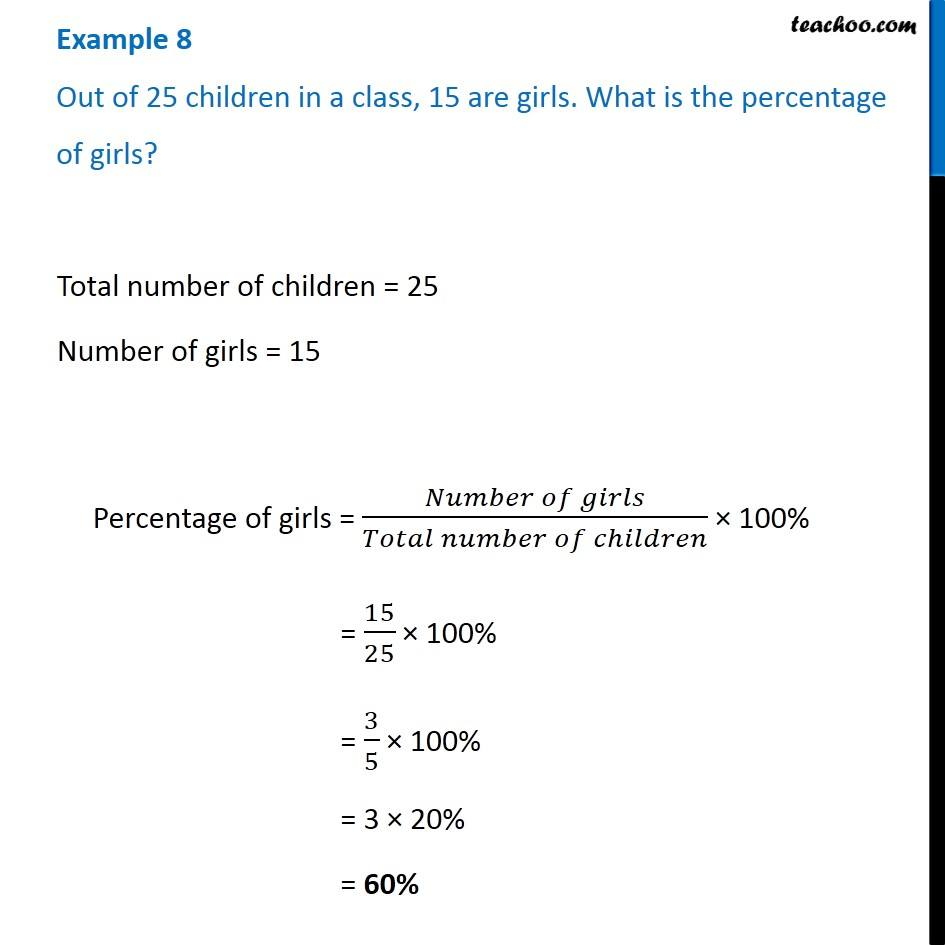Examples

Chapter 8 Class 7 Comparing Quantities
Serial order wise### Transcript

Example 8 Out of 25 children in a class, 15 are girls. What is the percentage of girls? Total number of children = 25 Number of girls = 15 Percentage of girls = (𝑁𝑢𝑚𝑏𝑒𝑟 𝑜𝑓 𝑔𝑖𝑟𝑙𝑠)/(𝑇𝑜𝑡𝑎𝑙 𝑛𝑢𝑚𝑏𝑒𝑟 𝑜𝑓 𝑐ℎ𝑖𝑙𝑑𝑟𝑒𝑛) × 100% = 15/25 × 100% = 3/5 × 100% = 3 × 20% = 60%